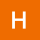Home
IT Knowledge
Inspiration
Languages
EN

# Java - generate set with 10 random unique numbers

3 points
Created by:halfera
321

## Generate set with 10 random unique numbers in Java.

``````int minVal = 50;
int maxVal = 100;
Set<Integer> unique = new HashSet<>();

while (unique.size() != 10) {
}

// [64, 65, 82, 68, 70, 88, 89, 60, 78, 95]
System.out.println(unique.toString());``````

Code flow:

1. Set random numbers minVal = 50 and maxVal = 100
2. Until set size is not 10 add new random int - so we execute while logic until HashSet.size() != 10
3. Generate random number between minVal = 50 and maxVal = 100
4. HashSet will add new number only if it is new, if the number already exists in the HashSet then it will be ignored.
5. Display results with sys out

To get the code for random min max long, float, double check post:

There are other ways to generate random integer in range min-max, look at this post: